Next: Example 5.3: Electric potential due Up: Electric Potential Previous: Example 5.1: Charge in a

## Example 5.2: Motion of an electron in an electric field

Question: An electron in a television set is accelerated from the cathode to the screen through a potential difference of +1000 V. The screen is 35 mm from the cathode. What is the net change in the potential energy of the electron during the acceleration process? How much work is done by the electric field in accelerating the electron? What is the speed of the electron when it strikes the screen?

Solution: Let call the cathode pointand the screen point. We are told that the potential difference between pointsandis +1000 V, soBy definition, the difference in electric potential energy of some chargeat pointsandis the product of the charge and the difference in electric potential between these points. Thus,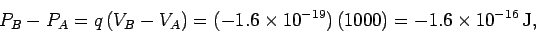since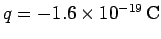for an electron. Note that the potential energy of the electron decreases as it is accelerated towards the screen. As we have seen, the electric potential energy of a charge is actually held in the surrounding electric field. Thus, a decrease in the potential energy of the charge corresponds to a reduction in the energy of the field. In this case, the energy of the field decreases because it does work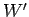on the charge. Clearly, the work done (i.e., energy lost) by the field equals the decrease in potential energy of the charge,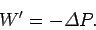Thus,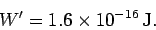The total energyof the electron is made up of two components--the electric potential energy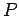, and the kinetic energy. Thus,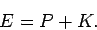Of course,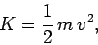where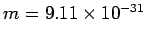kg is the mass of the electron, andits speed. By conservation of energy,is a constant of the motion, so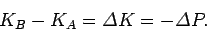In other words, the decrease in electric potential energy of the electron, as it is accelerated towards the screen, is offset by a corresponding increase in its kinetic energy. Assuming that the electron starts from rest (i.e.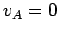), it follows that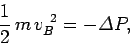or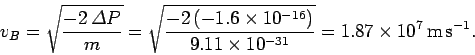Note that the distance between the cathode and the screen is immaterial in this problem. The final speed of the electron is entirely determined by its charge, its initial velocity, and the potential difference through which it is accelerated.Next: Example 5.3: Electric potential due Up: Electric Potential Previous: Example 5.1: Charge in a
Richard Fitzpatrick 2007-07-14